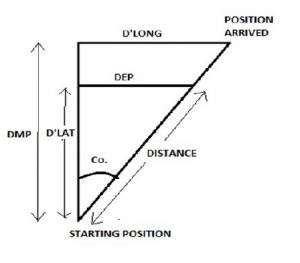Miscelleneous

# EXERCISE 3 — MERCATOR SAILING (Numerical Solution)

###### LONGITUDE
1.      24˚ 00.0’ N 074˚  15.0’ W 46˚ 00.0’ N 053˚  45.0’ W
2.      06˚ 00.0’ N 079˚  00.0’ W 38˚ 00.0’ S 179˚  00.0’ E
3.      40˚ 18.0’ N 100˚  20.0’ W 68˚ 00.0’ N 140˚  10.0’ E
4.      70˚ 20.0’ N 010˚  22.0’ W 52˚ 50.0’ N 009˚  45.0’ E
5.      32˚ 29.0’ S 064˚  00.0’ E 49˚ 50.0’ S 005˚  15.0’ E
###### NOTE:
• Where the distance exceeds 600 M,it is generally recommended to use Mercator sailing so that results are more accurate results obtained.
• To get DMP, apply same rule as for D’Lat i.e, same names subtract, different names add.
• To get distance, use plane sailing formula.
• In order to understand and solve such questions in a better and easy way, one must always draw the diagram.

SOLUTION:

###### D’LAT   22˚ 00.0’ N           D’LONG       020˚ 30.0’ W            DMP       1624.16

We know that:

TAN CO. = (D’LONG / DMP)
= (1230 / 1624.16)
COURSE = N 37˚ 08.23’ W

DISTANCE = (D’LAT × SEC CO.)
DIST. = 1655.81 M

COURSE = N 37˚ 08.23’ W   DIST. = 1655.81 M

###### D’LAT       44˚ 00.0’ S             D’LONG      102˚ 00.0’ W        DMP         2812.05

We know that:
TAN CO. = (D’LONG / DMP)
= (6120 / 2812.05)
COURSE = S65˚ 19.31’ W

DISTANCE =( D’LAT × SEC CO.)
DISTANCE  = 6323.03 M

COURSE = S65˚ 19.31’ W   DISTANCE  = 6323.03 M

###### D’LAT       27˚ 42.0’ N             D’LONG      119˚ 30.0’ W        DMP        2978.00

We know that:
TAN CO. = (D’LONG / DMP)
= (7170 /2978)
COURSE = N 67˚ 26.7’ W

DISTANCE = (D’LAT × SEC CO.)
DIST. = 4332.97 M

COURSE = N 67˚ 26.7’ W                   DIST. = 4332.97 M

###### D’LAT       17˚ 30.0’ S             D’LONG         020˚ 07.0’ E          DMP       2274.29

We know that:
TAN CO. = (D’LONG / DMP)
= (1207/2274.29)
COURSE = S27˚ 57.3’ E

DISTANCE = (D’LAT × SEC CO.)
DIST. = 1188.7 M

COURSE = S27˚ 57.3’ E         DIST. = 1188.7 M

###### D’LAT       17˚ 21.0’ S         D’LONG      058˚ 45.0’ W           DMP           1390.95

We know that:
TAN CO. = (D’LONG/DMP)
= (3525/1390.95)
COURSE = S 68˚ 28.00’ W

DISTANCE = D’LAT × SEC CO
DIST. = 2836.18 M

COURSE = S68˚ 28.00’ W                                            DIST. = 2836.18 M

###### DISTANCE
6.      36˚ 48.0’ N 085˚  53.0’ W 241˚ 1897 M
7.      06˚ 10.0’ S 176˚  47.0’ W 333˚ 4450 M
8.      38˚ 18.0’ S 005˚  11.0’ W 124˚ 3256 M
9.      44˚ 11.0’ N 140˚  20.0’ W 056˚ 2222 M
10.      18˚ 58.0’ N 072˚  52.0’ E 265˚ 7126 M#### Vikrant_sharma

•mojtaba says:
•Cj says: